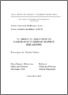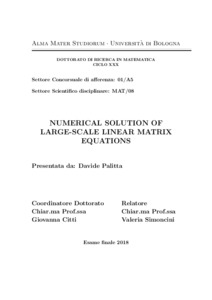# Numerical solution of large-scale linear matrix equations

Palitta, Davide (2018) Numerical solution of large-scale linear matrix equations, [Dissertation thesis], Alma Mater Studiorum Università di Bologna. Dottorato di ricerca in Matematica, 30 Ciclo. DOI 10.6092/unibo/amsdottorato/8342.
Documenti full-text disponibili:Anteprima
Documento PDF (English) - Richiede un lettore di PDF come Xpdf o Adobe Acrobat Reader
Disponibile con Licenza: Salvo eventuali più ampie autorizzazioni dell'autore, la tesi può essere liberamente consultata e può essere effettuato il salvataggio e la stampa di una copia per fini strettamente personali di studio, di ricerca e di insegnamento, con espresso divieto di qualunque utilizzo direttamente o indirettamente commerciale. Ogni altro diritto sul materiale è riservato.

## Abstract

We are interested in the numerical solution of large-scale linear matrix equations. In particular, due to their occurrence in many applications, we study the so-called Sylvester and Lyapunov equations. A characteristic aspect of the large-scale setting is that although data are sparse, the solution is in general dense so that storing it may be unfeasible. Therefore, it is necessary that the solution allows for a memory-saving approximation that can be cheaply stored. An extensive literature treats the case of the aforementioned equations with low-rank right-hand side. This assumption, together with certain hypotheses on the spectral distribution of the matrix coefficients, is a sufficient condition for proving a fast decay in the singular values of the solution. This decay motivates the search for a low-rank approximation so that only low-rank matrices are actually computed and stored remarkably reducing the storage demand. This is the task of the so-called low-rank methods and a large amount of work in this direction has been carried out in the last years. Projection methods have been shown to be among the most effective low-rank methods and in the first part of this thesis we propose some computational enhanchements of the classical algorithms. The case of equations with not necessarily low rank right-hand side has not been deeply analyzed so far and efficient methods are still lacking in the literature. In this thesis we aim to significantly contribute to this open problem by introducing solution methods for this kind of equations. In particular, we address the case when the coefficient matrices and the right-hand side are banded and we further generalize this structure considering quasiseparable data. In the last part of the thesis we study large-scale generalized Sylvester equations and, under some assumptions on the coefficient matrices, novel approximation spaces for their solution by projection are proposed.

Abstract
Tipologia del documento
Tesi di dottorato
Autore
Palitta, Davide
Supervisore
Dottorato di ricerca
Ciclo
30
Coordinatore
Settore disciplinare
Settore concorsuale
Parole chiave
Lyapunov equations, Sylvester equations, matrix equations
URN:NBN
DOI
10.6092/unibo/amsdottorato/8342
Data di discussione
16 Aprile 2018
URI